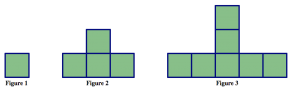$$\newcommand{\id}{\mathrm{id}}$$ $$\newcommand{\Span}{\mathrm{span}}$$ $$\newcommand{\kernel}{\mathrm{null}\,}$$ $$\newcommand{\range}{\mathrm{range}\,}$$ $$\newcommand{\RealPart}{\mathrm{Re}}$$ $$\newcommand{\ImaginaryPart}{\mathrm{Im}}$$ $$\newcommand{\Argument}{\mathrm{Arg}}$$ $$\newcommand{\norm}{\| #1 \|}$$ $$\newcommand{\inner}{\langle #1, #2 \rangle}$$ $$\newcommand{\Span}{\mathrm{span}}$$

5.4: Growing Patterns

•• Contributed by Michelle Manes
• Professor (Mathematics) at University of Hawaii

$$\newcommand{\vecs}{\overset { \rightharpoonup} {\mathbf{#1}} }$$

$$\newcommand{\vecd}{\overset{-\!-\!\rightharpoonup}{\vphantom{a}\smash {#1}}}$$

Here is a pattern made from square tiles.Think / Pair / Share

• Describe how you see this pattern growing. Be as specific as you can. Draw pictures and write an explanation to make your answer clear.
• Say as much as you can about this growing pattern. Can you draw pictures to extend the pattern?

Here are some pictures that students drew to describe how the pattern was growing.Ali's pictureMichael's pictureKelli's picture

Think / Pair / Share

Describe in words how each student saw the pattern growing. Use the students’ pictures above (or your own method of seeing the growing pattern) to answer the following questions:

• How many tiles would you need to build the 5th figure in the pattern?
• How many tiles would you need to build the 10th figure in the pattern?
• How can you compute the number of tiles in any figure in the pattern?

Problem 15

Hy saw the pattern in a different way from everyone else in class. Here’s what he drew:Hy's picture.

1. Describe in words how Hy saw the pattern grow.
2. How would Hy calculate the number of tiles needed to build the 10th figure in the pattern?
3. How would Hy calculate the number of tiles needed to build the 100th figure in the pattern?
4. How would Hy calculate the number of tiles needed to build any figure in the pattern?

The next few problems present several growing patterns made with tiles. For each problem you work on, do the following:

1. Describe in words and pictures how you see the pattern growing.
2. Calculate the number of tiles you would need to build the 10th figure in the pattern. Justify your answer based on how the pattern grows.
3. Calculate the number of tiles you would need to build the 100th figure in the pattern.
4. Describe how you can figure out the number of tiles in any figure in the pattern. Be sure to justify your answer based on how the pattern grows.
5. Could you make one of the figures in the pattern using exactly 25 tiles? If yes, which figure? If no, why not? Justify your answer.
6. Could you make one of the figures in the pattern using exactly 100 tiles? If yes, which figure? If no, why not? Justify your answer.

Problem 16Problem 17Problem 18Problem 19Problem 20